Precalculus : Determine the domain of a trigonometric function

Example Questions

Example Question #1 : Trigonometric Functions

Which of the following is the correct domain of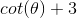, whererepresents an integer?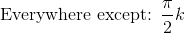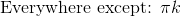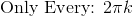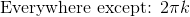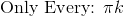Explanation:

The cotangent graph only has a period ofintervals and is most similar to the tangent graph.  The domain of cotangent exists everywhere except every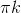value since an asymptote exists at those values in the domain.

The y-intercept of 3 shifts the cotangent graph up by three units, so this does not affect the domain.

Therefore, the graph exists everywhere except, whereis an integer.

Example Question #2 : Determine The Domain Of A Trigonometric Function

What is the domain of the following function?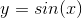Explanation:

All x values make the function work. Thus, making the domain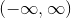. They're parentheses instead of brackets because parentheses are used when you can't actually use the specific value next to it. It is impossible to use infinity which makes parentheses appropriate. Brackets are used when you CAN use the specific value next to it.

Example Question #1 : Trigonometric Functions

What is the domain of the following function: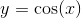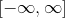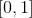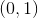Explanation:

All x values work for the function. Thus, making the domain all real numbers. Parentheses are required because you can never actually use the number infinity.

Example Question #2 : Trigonometric Functions

What is the domain of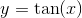.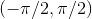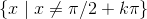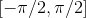Explanation:

If you look at a graph of the function, you can see that every curve has a vertical asymptote that repeats everyradians in the positive and negative x-direction, starting at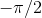radians.  Also, the curve has a length that stretchesradians which makes the domain.

Example Question #1 : Trigonometric Functions

What is the restriction of the domain of the function given by: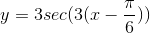For all the answer choices below,is any integer.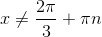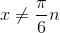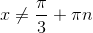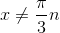Explanation: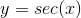has restrictions on its domain such that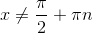, whereis any integer.

To determine the domain forwe equate the terms within the secant function and set them equal to the original domain restriction.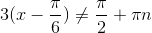Solving for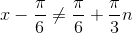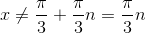The new domain restriction is:whereis an integer

All Precalculus Resources